# Transforming shapes with matrices

### Transforming shapes with matrices

In this section, we will learn how to transform shapes with matrices. Instead of one column vector, we are going to have multiple vertices which create a shape. What we can do to this shape is use the transformation matrix to change the length and size of that shape. To do this computation, we merge all the vertices into one matrix and then multiply it with the transformation matrix. Doing so will give us another matrix. We will then take a look at each transformed vertices separately in the matrix to see the new transformed shape. Note that transforming the shape does not change the number of sides. We will take a look at some questions which involve transforming shapes, and then graph them to notice the changes between the normal shape and the transformed shape.

#### Lessons

Let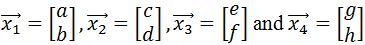be vertices of a square and $T$ be a transformation matrix.
Then we can transform the square by combining the vertices into a matrix (denoted by $A$), and multiply it by the transformation matrix $T$. In other words,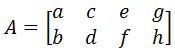And $TA$ is the transformed square.
Of course, this idea can also apply to other shapes other than squares.
• Introduction
Transforming shapes with matrices overview

• 1.
Finding the Transformed Polygons
Apply the transformation matrix $T$ to the following vertices to find the transformed vertices:
a)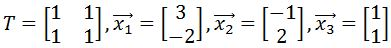b)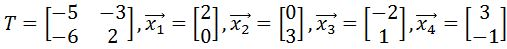c)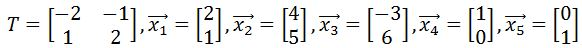• 2.
Graphing the Transformed Polygon
Plot the vertices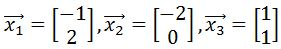on the graph. Then apply the transformation matrix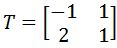, to the vertices to find the transformed polygon, and then plot the transformed polygon on the graph.

• 3.
Plot the vertices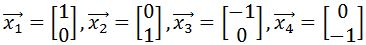on the graph. Then apply the transformation matrix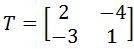, to the vertices to find the transformed polygon, and then plot the transformed polygon on the graph.

• 4.
Plot the vertices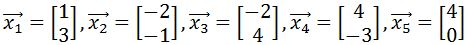on the graph. Then apply the transformation matrix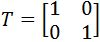, to the vertices to find the transformed polygon, and then plot the transformed polygon on the graph.